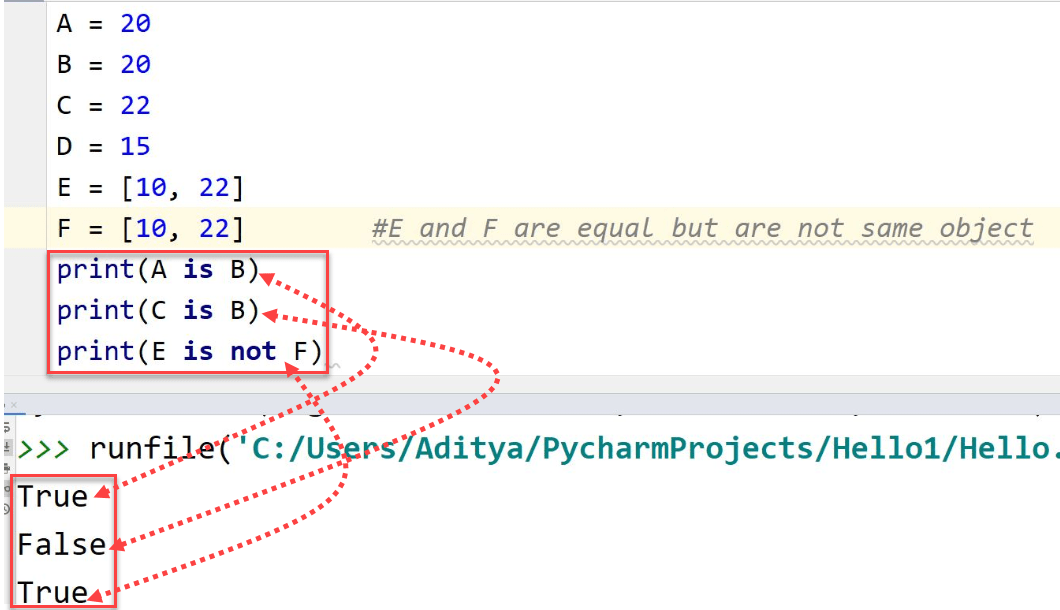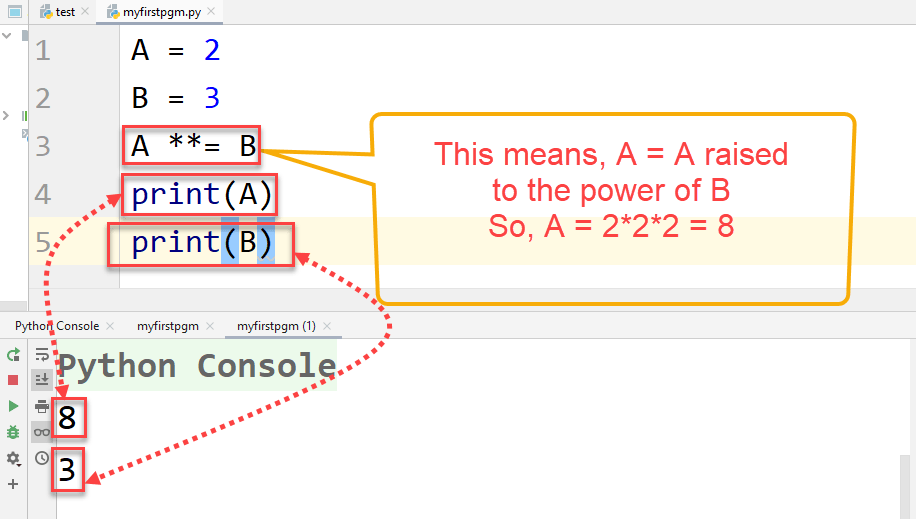# Python Operators

Operators are used for operations on various variables and constants. In another words, each Operator can hold two values on each side and it returns a value and this value depends on the type of Operator used, e.g., 10*2 = 20 (Here the sign * is an Operator), but the value will change if we use a “+” operator here. There are many types of different operators in Python that are supported, let’s have a look: –

• Arithmetic Operators
• Logical Operators
• Bitwise Operators
• Identity Operators
• Membership Operators
• Assignment Operators
• Relational Operators (Also known as Comparison Operators)

We are going to take a look at each type one by one.

## Arithmetic Operators

Arithmetic operators helps to carry out mathematical operations are called Arithmetic Operators, e.g., +  (Addition), – (Subtraction), * (Multiplication), etc.  Here is a table of all Arithmetic Operators that are used in Python: –

Let’s see how these operators are used in Python –

Arithmetic operators
Operator Name Use of the Operator
Addition, + This operator add the two or more values or variables.
Subtraction, - This operand subtracts the value of the right hand side from the value present in the left hand side.
Multiplication, * Multiplies 2 or more variables.
Exponent, ** The right-side value becomes the exponent of the value that is on the left side.
Modulus, % Returns the remainder of the division.
Floor Division, // This operator divides the left side value by the value on the right side and then returns the smallest and closest integer, meaning that output will always be an integer and not a float.

## Example## Logical Operators

Logical Operators are very useful in codes where there are test cases present. Logical Operators include “and”, “or”, and “not”. Like most of the Operators, Logical Operators also have two operands except “not”, it can only perform operations only on a single operator. This is how these Operators work: –

Operator Truth Tables

Let’s assume there are two inputs namely “X” and “Y” and an output named as “Z”.

• Truth table for “and” operator

XYZ
FalseFalseFalse
FalseTrueFalse
TrueFalseFalse
TrueTrueTrue
• Truth Table for “or” operator

XYZ
FalseFalseFalse
FalseTrueTrue
TrueFalseTrue
TrueTrueTrue
• Truth Table for “not” operator

XNot X
FalseTrue
TrueFalse

These operators are useful when their conditions present in a code. False means that the conditions are not satisfied and the code has to stop itself from executing and True means that the condition(s) has been satisfied and the code can continue its execution. Let’s take an example based on the truth tables above –

## Example## Bitwise Operators

These operators are used to compare two binary digits. As we already know, 1 means True and 0 means False. Bitwise operators treat the operand values as binary digits, and the operation on binary numbers is performed only on one bit per time from both operands (if the operator has two operands), hence the name.

Logical operator OR
Operator Name and Number of Operands Description
& AND (Two Operands) Sets each bit to 1 if both bits are 1
| OR (Two Operands) Sets each bit to 1 if either bit is 1
~ NOT (One Operand) Calculates ones’ complement of the bit.
^ XOR (Two Operands) Sets each bit to 1 if only one bit is 1
>> Right Shift (Two Operands) Shifts bytes to the right side and adds zeros to the left side. Number of zeros is equal to the right-side operand.
<< Left Shift (Two Operand) Shifts bytes to the left side and adds zeros to the right side. Number of zeros is equal to the left side operand

## Example## Identity Operators

Identity operators are used to check whether two operands are pointing to the same memory location or not. If two operands are equal, this does not mean that they also belong to the same object and refer to the same memory location.

Identity operators
Operator Description
is It will show True in the output If both operands are identical objects
is not It will show True in the output if the operands are unidentical objects.

## Example## Membership Operators

These operators are used to check whether an operand is present in a Sequence or not. The sequence can be List, String, etc.

Membership operators
Operator Description
in This will return True if the operand is present in the Sequence
not in This will return True if the operand is not present in the Sequence.

## Example## Assignment Operators

These operators are used to assign values to some variable.

Membership operators
Operator and its Name Description
=, Equal This operator transfers the value of the right operand to the left
+=, Add AND This adds the operand on the right hand side to the left-side operand and assigns the final result to the left hand side operand.
-=, Subtract AND This subtracts the right hand side operand from the left hand side operand and assigns the final result to the left hand side operand.
/=, Divide AND It performs the divides the left operand by the left operand and assigns the final result to the left side operand.
*=, Multiply AND It multiplies both the operands and the final value is assigned to the left hand side operand.
//=, Floor Division AND It performs normal division first and then converts the float integer to the smallest and the closest integer and then assigns this value to the left hand side operand.
**=. Exponent AND This operator assigns the final value of the exponent calculation to the left hand side operand.
%=, Modulus AND It calculates and assigns the remainder of the division to the left hand side operator.

## Example## Relational/Comparison Operators

Using Relational operators, you can compare two values. They can either return True or False depending upon the test case.

Relation/Comparison operators
Operator and its Name Syntax
==, Equal x == y
>, Greater than x > y
<, Smaller than x < y
!=, Not Equal x != y
>=, Greater than or Equal to x >= y
<=, Smaller than or Equal to x <= y

## Example